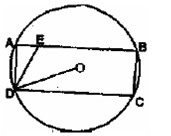In the figure below (not drawn to scale), rectangle ABCD. Is inscribed in the circle with centre at O. The length of side AB is greater than that of side BC. The ratio of the area of the circle to the area of the rectangle ABCD is π:√3 The line segment DE intersects AB at E such that angle ODC = angle ADE. What is the ratio AE:AD